you are here:/ News & Insights / Engineering Advantage Blog / How to Include the Effect of Stress Relaxation When Simulating Creep Response

# How to Include the Effect of Stress Relaxation When Simulating Creep ResponseNovember 7, 2014 By: Michael Bak

Question:  When performing a finite element analysis that includes the simulation of creep response, what additional information must be entered to include the effect of stress relaxation?

Answer:  Relax!  No additional information is required; the effect of relaxation is automatically included when modeling creep.

To understand how creep and relaxation are modeled in finite element analysis, the terms "creep" and "stress relaxation" will be defined.  Creep is the progressive deformation of a material at constant stress.  For engineering metals, creep is usually important at elevated temperatures (e.g. above 30% of the melting point in absolute temperature units) and under high stress conditions.  For plastic materials, creep can occur at room temperature.  Stress relaxation is the decrease of stress in a material under constant strain.

Creep can be included in a finite element analysis in several ways.  The most common approach is to define a creep law, which defines the creep strain rate of accumulation as a function of the current stress, strain, time and temperature.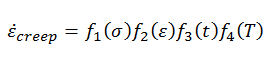Creep tests are used to obtain data for a given material, and then nonlinear regression curve-fitting is used to fit the data to the creep law.  When performing a creep analysis, the basic assumption is that the total strain is determined by independently calculating the various strain components, i.e. elastic strain, plastic strain, and creep strain, and then adding them together, or in equation form: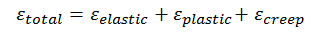Note that there are other analytical approaches to modeling creep, such as defining viscoplastic material models.  These models treat inelastic strains in a strongly-coupled manner and will be discussed in a future blog post.

Following the advice of using simple test cases, consider a model where we apply a uniform static tension load that will produce a stress of 2000 psi, then model the time-dependent response by including a creep law and looking at the response over time.  Since a constant load is applied, and the model is free to deform, the stress will stay constant as will the elastic strain, but the creep strain will accumulate and result in increased total strain, as represented in the following figure.  This case represents a pure creep response.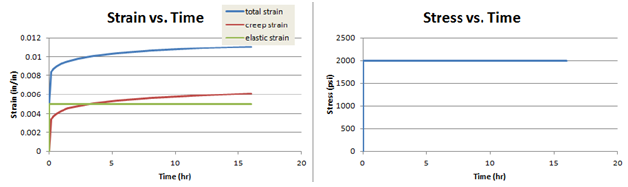Using the exact same model with the exact same creep law, the loading is changed from an applied load to an applied displacement that will produce the same stress magnitude of 2000 psi. After the displacement is applied, it is held fixed in that position and the time-dependent response is calculated.  For this case, since the total strain is fixed, the increase in the creep strain accumulation will result in a reduction in the elastic strain (since the sum of elastic strain and creep strain equals the total strain). Since the stress is based on the elastic strain, the reduction in elastic strain results in a reduction in stress.  This behavior, captured in the figure below, represents pure stress relaxation.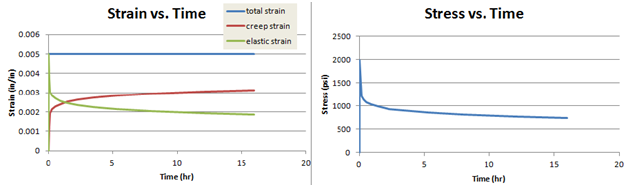Relaxation test data is typically not as common as creep data.  Fortunately, for most engineering materials, creep data give a good approximation of the relaxation phenomenon, and the described approach is a standard modeling technique.

So what happens in the general case of a more complex finite element analysis?  For every point in the model that is accumulating creep strain, the response will either predict pure creep, pure stress relaxation, or most likely, a combination of both.  For example, consider the following plot which contains the total, elastic, plastic and creep strains, as well as the corresponding stress, at a point taken from a more complex finite element solution.  The plot shows that as the creep strain accumulates over time, the total strain is increasing, and thus creep behavior is predicted.  However, the creep strain is increasing faster than the total strain.  Thus not all of the creep strain is being added to the total strain.  In other words, the creep strain accumulated at this point cannot all be translated into increased deformation, and thus the elastic strain is reduced over time as well, causing some stress relaxation.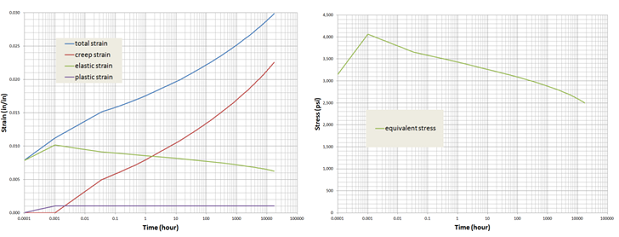Thus, the accumulation of creep strain provides both creep and relaxation response, based on the local conditions at that particular location in the model.  If you have any thoughts or comments on this, even if they are a bit creepy, let us know!